# Civil Engineering - UPSC Civil Service Exam Questions

6.
A column section as indicated in the given figure is loaded with a concentrated load at a point 'F' so as to produce maximum bending stress due to eccentricities about xx axis and yy axis as 5 t/m2 and 8 t/m2 respectively. If the direct stress due to loading is 15 t/m2 (compressive), then the intensity of resultant stress at the corner 'B' of the column section is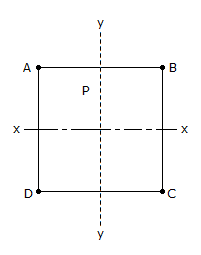2 t/m (compressive)
12 t/m (compressive)
18 t/m2 (tensile)
28 t/m2 (compressive)
Explanation:
No answer description is available. Let's discuss.

7.
A vertical gate 6 m x 6 m holds water on one side with the free surface at its top. The moment about the bottom edge of the gate of the water force will be (v is the specific weight of water)
18 v
36 v
72 v
216 v
Explanation:
No answer description is available. Let's discuss.

8.
A beam of square beam is given by section 'a' is used with its diagonal horizontal. The modulus of section of the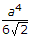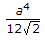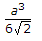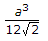Explanation:
No answer description is available. Let's discuss.

9.
An isosceles triangular plate of base 3 m and altitude 3 m is immersed vertically in an oil of specific gravity 0.8. The base of the plate coincides with the free surface of oil. The centre of pressure will lie at a distance of (from free surface)
2.5 m
2 m
1.5 m
1 m
Explanation:
No answer description is available. Let's discuss.

10.
Consider the following statements regarding the swedish circle method of analysing stability of slopes :
1. It is a general method of analysing stability of slopes.
2. It satisfied only the overall moment equation of equilibirum.
3. It considers the forces action on the sides of individual slices.
4. It gives a factor of safety which are on the safeside.
Of the statements :
1, 2 and 3 are correct
1, 2 and 4 are correct
2, 3 and 4 are correct
1, 3 and 4 are correct